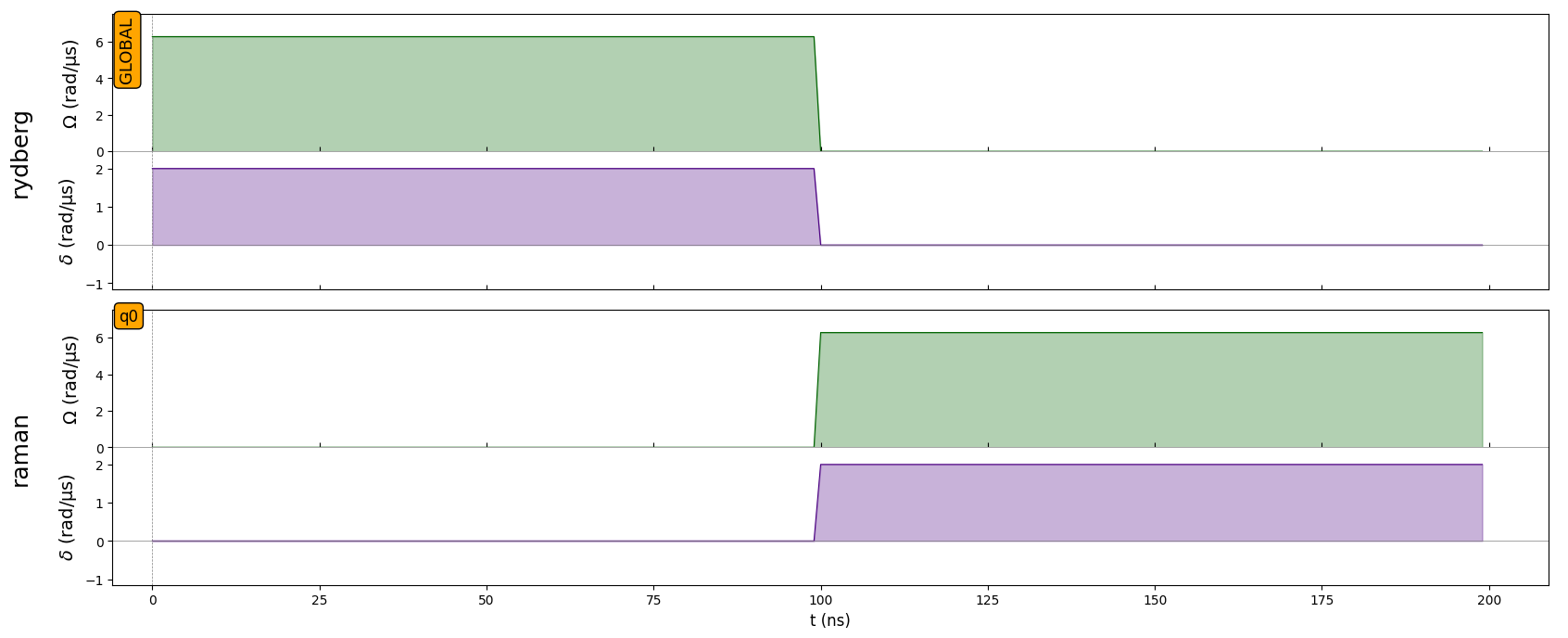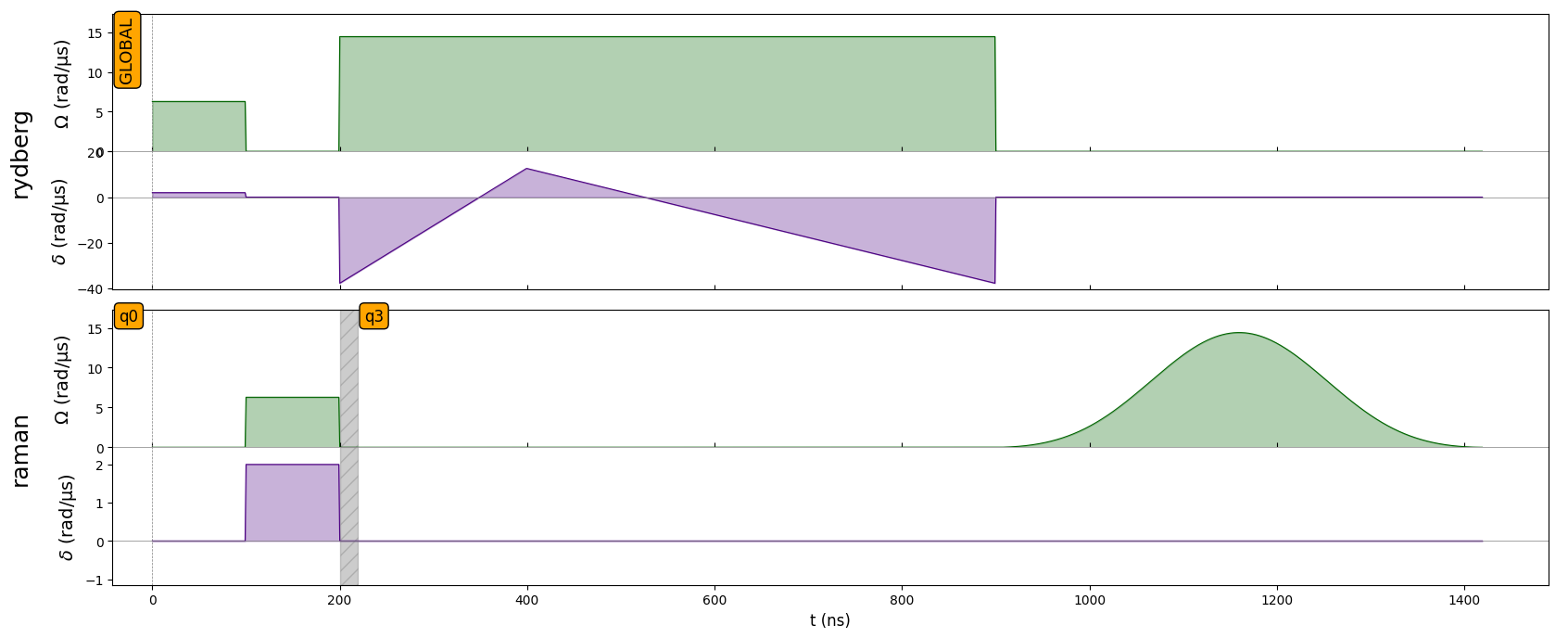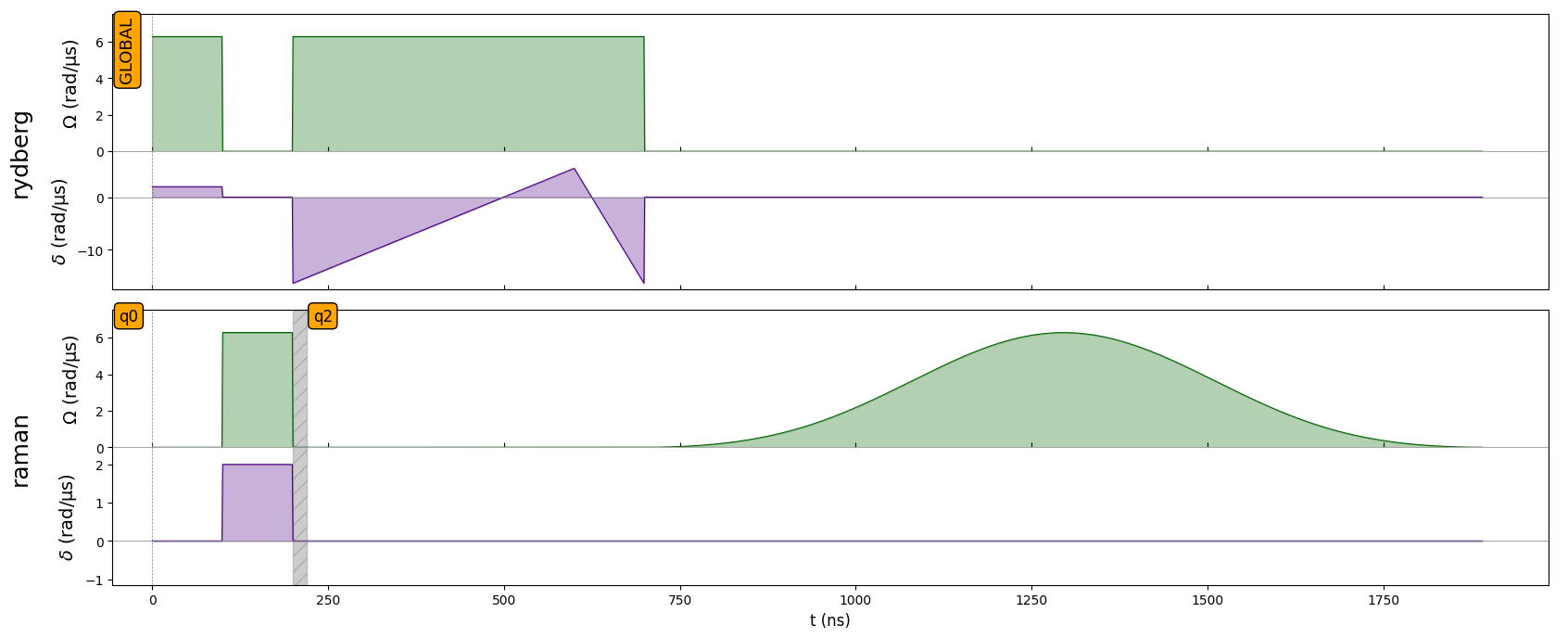# Parametrized Sequences

:

import numpy as np
import pulser
from pulser import Pulse, Sequence, Register
from pulser.waveforms import RampWaveform, BlackmanWaveform, CompositeWaveform
from pulser.devices import DigitalAnalogDevice


From simple sweeps to variational quantum algorithms, it is often the case that one wants to try out multiple pulse sequences that vary only in a few parameters. For this effect, the ability to make a Sequence parametrized was developed.

A parametrized Sequence can be used just like a “regular” Sequence, with a few key differences. Initialization and channel declaration, for example, don’t change at all:

:

reg = Register.square(2, prefix="q", spacing=6)
seq = Sequence(reg, DigitalAnalogDevice)
seq.declare_channel("rydberg", "rydberg_global")
seq.declare_channel("raman", "raman_local")


## Variables and Parametrized Objects

The defining characteristic of a parametrized Sequence is its use of variables. These variables are declared within a Sequence, by calling:

:

Omega_max = seq.declare_variable("Omega_max")
ts = seq.declare_variable("ts", size=2, dtype=int)
last_target = seq.declare_variable("last_target", dtype=int)


The returned Omega_max, ts and last_target objects are of type Variable, and are defined by their name, size and data type. In this case, Omega_max is a single variable with dtype=float (the default), ts is an array of two int values and last_target is an int.

These returned Variable objects support simple arithmetic operations (when applicable) and, when of size > 1, even item indexing. Take the following examples:

:

t_rise, t_fall = ts  # Unpacking is possible too
U = Omega_max / 2.3
delta_0 = -6 * U
delta_f = 2 * U
t_sweep = (delta_f - delta_0) / (2 * np.pi * 10) * 1000


Both the original Variables and the results of these operations serve as valid inputs for Waveforms, Pulses or Sequence-building instructions. We can take Omega_max as an argument for a waveform:

:

pi_wf = BlackmanWaveform.from_max_val(Omega_max, np.pi)


or use derived quantities, like t_rise, t_fall, delta_0 and delta_f:

:

rise_wf = RampWaveform(t_rise, delta_0, delta_f)
fall_wf = RampWaveform(t_fall, delta_f, delta_0)
rise_fall_wf = CompositeWaveform(rise_wf, fall_wf)


These waveforms are parametrized objects, so usual attributes like duration or samples are not available, as they depend on the values of the underlying variables. Nonetheless, they can be used as regular waveforms when creating Pulses, which will consequently be parametrized too.

:

pi_pulse = Pulse.ConstantDetuning(pi_wf, 0, 0)
rise_fall = Pulse.ConstantAmplitude(Omega_max, rise_fall_wf, 0)


## Constructing the Sequence

Upon initialization, a Sequence is, by default, not parametrized. We can check this by calling:

:

seq.is_parametrized()

:

False


While it is not parametrized, it is just a normal sequence. We can do the usual stuff, like targeting a local channel, adding regular pulses, or plotting the sequence:

:

generic_pulse = Pulse.ConstantPulse(100, 2 * np.pi, 2, 0.0)
seq.target("q0", "raman")
seq.draw()The Sequence becomes parametrized at the moment a parametrized object or variable is given to a sequence-building instruction. For example:

:

seq.target_index(last_target, "raman")
seq.is_parametrized()

:

True


From this point onward, functionalities like drawing are no longer available, because the instructions start being stored instead of executed on the fly. We can still check the current state of a parametrized sequence by printing it:

:

print(seq)

Prelude
-------
Channel: rydberg
t: 0 | Initial targets: q0, q1, q2, q3 | Phase Reference: 0.0
t: 0->100 | Pulse(Amp=6.28 rad/µs, Detuning=2 rad/µs, Phase=0) | Targets: q0, q1, q2, q3

Channel: raman
t: 0 | Initial targets: q0 | Phase Reference: 0.0
t: 0->100 | Delay

Stored calls
------------

1. target_index(last_target, raman)


Naturally, we can also add the parametrized pulses we previously created:

:

seq.add(rise_fall, "rydberg")


## Building

Once we’re happy with our parametrized sequence, the last step is to build it into a regular sequence. For that, we call the Sequence.build() method, in which we must attribute values for all the declared variables:

:

built_seq = seq.build(
Omega_max=2.3 * 2 * np.pi,
ts=[200, 500],
last_target=reg.find_indices(["q3"]),
)
built_seq.draw()

/home/docs/checkouts/readthedocs.org/user_builds/pulser/envs/latest/lib/python3.9/site-packages/pulser/sequence/sequence.py:1319: UserWarning: A duration of 519 ns is not a multiple of the channel's clock period (4 ns). It was rounded up to 520 ns.:

alt_seq = seq.build(